### Home > PC > Chapter 1 > Lesson 1.1.4 > Problem1-54

1-54.
1. Give the opposite and reciprocal of each expression without doing any arithmetic. If the given expression involves fractions, give the answer with positive exponents. Homework Help ✎

 Example: 32 Solution: Opposite = −32 Reciprocal = 3−2 or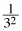Example: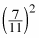Solution: Opposite = −Reciprocal =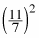1. 54

2. 3−5

3. 11−6

4.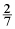5.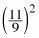6.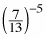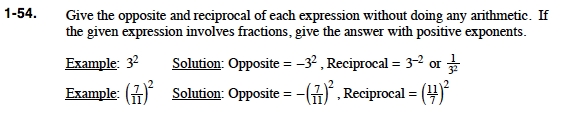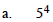$\text{Opposite} = -5^4$

$\text{Reciprocal} = -5^4=\frac{1}{5^4}$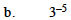$\text{Opposite} = -3^{-5}$

$\text{Reciprocal} = 3^5$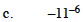$\text{Opposite} = 11^{-6}$

$\text{Reciprocal} = -11^6$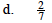$\text{Opposite} = -\frac{2}{7}$

$\text{Reciprocal} =\frac{7}{2}$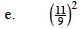$\text{Opposite} = -\left(\frac{11}{9}\right)^2$

$\text{Reciprocal} = \left(\frac{11}{9}\right)^{-2}= \left(\frac{9}{11}\right)^{2}$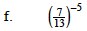$\text{Opposite} = -\left(\frac{7}{13}\right)^{-5}$

$=\left(-\frac{7}{13}\right)^{-5}$

$\text{Reciprocal} = \left(\frac{7}{13}\right)^{5}$